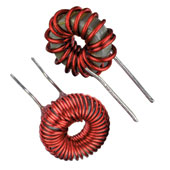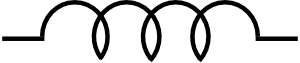Inductors are passive electrical component and they store energy in magnetic field created by an electric current whereas capacitors store energy in electric field.

A inductor can be simply created by wrapping wire around an object. Ferromagnetic core,solid core of copper or iron and steel core are possible objects. When electric current passes through the conductor ( wire around core) , magnetic flux is created.

If a change in electic current, magnetic flux is also changed. Consequently, by Faraday's law, electro motive force (EMF) is generated and it opposes the change in current.An Inductor's ability to store magnetic energy is known as Inductance (L) and measured in the SI unit of henries (H). Inductance is a measure of the amount of EMF generated per unit change in current. An inductor's differential equation shows relationship between time varying voltage V(t) across it and time varying current i(t) through it.

##### V (t) = L di (t) / dt

where V(t) is sinusoidal votage induced and i(t) is sinusoidal alternating current.

The symbol of a inductor is drawn as belowIn AC circuits, resisting current flow is measured in impedance and impedance is a combination of resistance and reactance. Reactance in an inductor can be calculated as

##### R (L) = 2 π F L

where F is frequency in Hertz and L is inductance of inductor coil. Inductors are used extensively in analog circuits and signal processing. It is used in tank circuits along with capacitors. Transformers are created by wrapping two or more coils around a core object.